Hostname: page-component-cd4964975-598jt Total loading time: 0 Render date: 2023-03-29T22:38:56.673Z Has data issue: true Feature Flags: { "useRatesEcommerce": false } hasContentIssue true

# The SL(2, C) Casson Invariant for Knots and the Â-polynomial

Published online by Cambridge University Press:  20 November 2018

## Abstract

HTML view is not available for this content. However, as you have access to this content, a full PDF is available via the ‘Save PDF’ action button.

In this paper, we extend the definition of the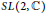$SL\left( 2,\,\mathbb{C} \right)$ Casson invariant to arbitrary knots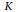$K$ in integral homology 3-spheres and relate it to the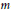$m$-degree of the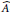$\widehat{A}$-polynomial of$K$. We prove a product formula for the$\widehat{A}$-polynomial of the connected sum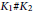${{K}_{1}}\#{{K}_{2}}$ of two knots in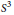${{S}^{3}}$ and deduce additivity of the$SL\left( 2,\,\mathbb{C} \right)$ Casson knot invariant under connected sums for a large class of knots in${{S}^{3}}$. We also present an example of a nontrivial knot$K$ in${{S}^{3}}$ with trivial$\widehat{A}$-polynomial and trivial$SL\left( 2,\,\mathbb{C} \right)$ Casson knot invariant, showing that neither of these invariants detect the unknot.

## Keywords

Type
Research Article
Information
Canadian Journal of Mathematics , 01 February 2016 , pp. 3 - 23

## References

 Boden, H. U., Nontriviality of the M-degree of the A-polynomial. Proc. Amer. Math. Soc. 142(2014), no. 6, 21732177.http://dx.doi.org/10.1090/S0002-9939-2014-11936-8 CrossRefGoogle Scholar
 Boden, H. U. and Curtis, C. L., The SL2(ℂ) Casson invariant for Seifert fibered homology spheres and surgeries on twist knots. J. Knot Theory Ramific. 15(2006), no. 7, 813837.http://dx.doi.org/10.1142/S0218216506004762 CrossRefGoogle Scholar
 Boden, H.U and Curtis, C.L, Splicing and the SL2(ℂ) Casson invariant. Proc. Amer. Math. Soc. 136(2008), no. 7, 26152623.http://dx.doi.org/10.1090/S0002-9939-08-09233-2 CrossRefGoogle Scholar
 Boden, H. U. and Curtis, C. L., The SL(2, ℂ) Casson invariant for for Dehn surgeries on two-bridge knots. Algebr. Geom. Topol. 12(2012), no. 4, 20952126.http://dx.doi.org/10.2140/agt.2012.12.2095 CrossRefGoogle Scholar
 Boyer, S. and Zhang, X., A proof of the finite filling conjecture. J. Differential Geom. 59(2001), 87176.CrossRefGoogle Scholar
 Chesebro, E., Closed surfaces and character varieties. Algebr. Geom. Topol. 13(2013), no. 4, 20012037.http://dx.doi.org/10.2140/agt.2013.13.2001 CrossRefGoogle Scholar
 Cooper, D., Culler, M., Gillet, H., Long, D. D., and Shalen, P. B., Plane curves associated to character varieties of 3-manifolds. Invent. Math. 118(1994), no. 1, 4784.http://dx.doi.org/10.1007/BF01231526 CrossRefGoogle Scholar
 Cooper, D. and Long, D. D., Remarks on the A-polynomial of a knot. J. Knot Theory Ramifications 5(1996), no. 5, 609628.http://dx.doi.org/10.1142/S0218216596000357 CrossRefGoogle Scholar
 Cooper, D. and Long, D. D., The A-polynomial has ones in the corners. Bull. Lond. Math. Soc. 29(1997), no. 2, 231238.http://dx.doi.Org/10.1112/SOO24609396002251 CrossRefGoogle Scholar
 Culler, M., C.|McA. Gordon, Luecke, J., and Shalen, P. B., Dehn surgery on knots. Ann. of Math. 125(1987), no. 2, 237300.http://dx.doi.Org/10.2307/1971311 CrossRefGoogle Scholar
 Culler, M. and Shalen, P. B., Varieties of group representations and splittings of 3-manifolds. Ann. of Math. 117(1983), no. 1, 109146.http://dx.doi.Org/10.2307/2006973 CrossRefGoogle Scholar
 Curtis, C. L., An intersection theory count of the SL(2,ℂ) -representations of the fundamental group of a 3-manifold. Topology 40(2001), 773787.http://dx.doi.Org/10.1016/S0040-9383(99)00083-X CrossRefGoogle Scholar
 Curtis, C. L., Erratum to “An intersection theory count of the SL(2,ℂ) - representations of the fundamental group of a 3-manifold”. Topology 42(2003), no. 4, 929.http://dx.doi.Org/10.1016/S0040-9383(02)00101-5 CrossRefGoogle Scholar
 Fulton, W., Algebraic curves. An introduction to algebraic geometry. Notes written with the collaboration of Richard Weiss, Mathematics Lecture Notes Series, W. A. Benjamin, New York, 1969.Google Scholar
 Johnson, D. and Millson, J., Deformation spaces associated to compact hyperbolic manifolds. In: Discrete groups in geometry and analysis (New Haven, Conn., 1984), Progr. Math., 67, Birkhäuser Boston, 1987, pp. 48106.CrossRefGoogle Scholar
 Kapovich, M. and Millson, J., On representation varieties of 3-manifold groups. arxiv:1303.2347Google Scholar
 Klassen, E. P., Representations in SU (2) of the fundamental groups of the Whitehead link and of doubled knots. Forum Math. 5(1993), 93109.http://dx.doi.Org/10.1515/form.1993.5.93 CrossRefGoogle Scholar
 Le, T. L. K, Varieties of representations and their cohomology-jump subvarieties for knot groups. (Russian) Mat. Sb. 184(1993), no. 2, 57–82; translation in: Russian Acad. Sci. Sb. Math.) 78(1994), no. 1, 187209.http://dx.doi.org/10.1070/SM1994v078n01ABEH003464 Google Scholar
 Le, T. K. T. and Tran, A. T., On the A] conjecture for knots. http://arxiv:1111.5258v4Google Scholar
 Marché, J., The skein module of torus knots. Quantum Topol. 1(2010), no. 4, 413421.http://dx.doi.Org/10.4171/QT/11 CrossRefGoogle Scholar
 Shafarevich, I. R., Basic algebraic geometry I. In: Varieties in protective space. Second edition. Springer-Verlag, Berlin, 1994.Google Scholar
 Sikora, A. S., Character varieties. Trans. Amer. Math. Soc. 364(2012), no. 10, 51735208.http://dx.doi.org/10.1090/S0002-9947-2012-05448-1 CrossRefGoogle Scholar
 Tran, A. T., The universal character ring of the (−2, 2m + 1, 2n)-pretzel link. Internat. J. Math. 24(2013), no. 8, 1350063.http://dx.doi.org/10.1142/S0129167X13500638 CrossRefGoogle Scholar